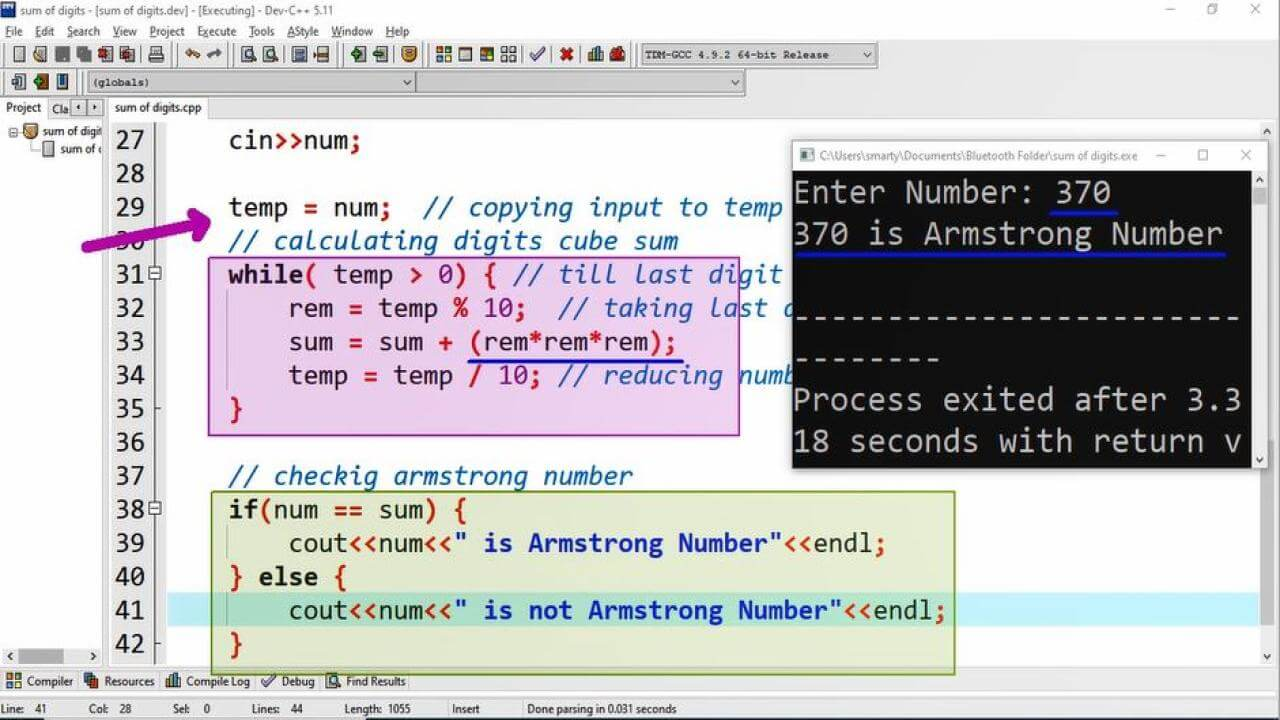# Armstrong Number Program Example using CPP

## Source Code:

#include <iostream>

// using standard namespace
using namespace std;

/*
If digits cube sum is equal
to the number itself then
number is called Armstrong Number
Eg:
371 = 3x3x3 + 7x7x7 + 1x1x1
*/

int main(int argc, char** argv) {

// Variable Declarations
int num;  // used to take input from user
int rem;  // used to store remainder of number
int temp; // This will hold copy of input

// variable initialization
int sum = 0;  // initializing with addition identity

// Input statement
cout<<"Enter Number: ";
cin>>num;

temp = num;  // copying input to temp
// calculating digits cube sum
while( temp > 0) { // till last digit
rem = temp % 10;  // taking last digit
sum = sum + (rem*rem*rem);  // Taking cube
temp = temp / 10; // reducing number by 1 digit
}

// checkig armstrong number
if(num == sum) {
cout<<num<<" is Armstrong Number"<<endl;
} else {
cout<<num<<" is not Armstrong Number"<<endl;
}
return 0;
}

## Output:## Working:

This program example input a number using cin statement and then check whether the given number is armstrong number or not. For this purpose the following statements are executed in while loop.

1. If number is greater than 0
2. Take remainder of number by 10
3. Calculate cube of remainder
4. Sum all cubes of digits
5. Repeat from step 1

In declaration statements we also declare an extra variable named as temp. This variable is only used to store an additional copy of input data. Because in processing number for finding cube of digits the number will goes to zero. To read about complete detail we can also learn from the Sum of Digits. This will help to understand the process of getting digits from numbers.

Finally we compare the calculated sum with orignal variable num using if else statement. If these two are equal then we print Armstrong otherwise not armstrong

## Example of Armstrong Numbers:

Some example numbers tested using this algorithm are 1, 153, 370, 371, 407,